NEET  >  Test: Free Body - 2

# Test: Free Body - 2

Test Description

## 10 Questions MCQ Test Physics Class 11 | Test: Free Body - 2

Test: Free Body - 2 for NEET 2022 is part of Physics Class 11 preparation. The Test: Free Body - 2 questions and answers have been prepared according to the NEET exam syllabus.The Test: Free Body - 2 MCQs are made for NEET 2022 Exam. Find important definitions, questions, notes, meanings, examples, exercises, MCQs and online tests for Test: Free Body - 2 below.
Solutions of Test: Free Body - 2 questions in English are available as part of our Physics Class 11 for NEET & Test: Free Body - 2 solutions in Hindi for Physics Class 11 course. Download more important topics, notes, lectures and mock test series for NEET Exam by signing up for free. Attempt Test: Free Body - 2 | 10 questions in 10 minutes | Mock test for NEET preparation | Free important questions MCQ to study Physics Class 11 for NEET Exam | Download free PDF with solutions
 1 Crore+ students have signed up on EduRev. Have you?
Test: Free Body - 2 - Question 1

### Free body diagram of a situation is shown below. The net force is known, however, the magnitude of few of the forces is not known. The magnitude of the unknown forces C and D will be: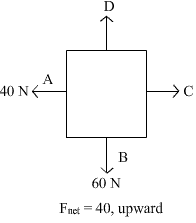Detailed Solution for Test: Free Body - 2 - Question 1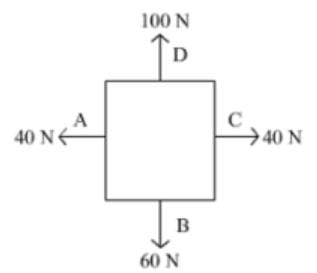Force on A’ and B’ is 40 N and 60 N respectively. Net force of the situation is 40 N upward. So to balance the force on A’ a force of 40 N has to apply on C’. Similarly a force of 100 N is also be applied to get the net force of 40 N in upward direction.

Test: Free Body - 2 - Question 2

### If a block moving up at an inclined plane with θ =30° with a velocity 5 m/s , stops after 0.5 sec , then coefficient of friction is:

Detailed Solution for Test: Free Body - 2 - Question 2

The initial velocity is 5 m/s and the block stops after ½ sec,
Hence the net negative acceleration is 10 m/s2
The net force active downwards parallel the plane is mg sin 30° + kmg cos 30°
Hence we get a = g ( sin 30° + kcos 30°) = 10
Thus we get  sin 30° + kcos 30° = 1
k = sec 30° - tan 30°
= 2/1.73 - 1/1.73
= 1/1.73
= 0.6

Test: Free Body - 2 - Question 3

### A person is sliding an iron box across the floor. Its free body diagram will be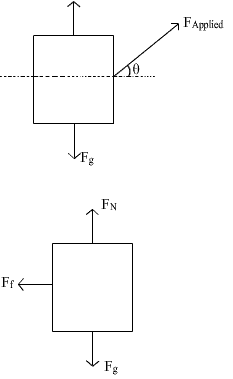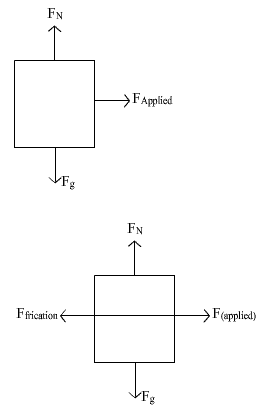Detailed Solution for Test: Free Body - 2 - Question 3

In the given condition, we get that a component of applied force acts parallel to force rightwards and one is perpendicular. Also the weight, normal and friction (leftwards) would also act upon it
Hence the correct Ans is D.

Test: Free Body - 2 - Question 4

Free body diagram of a box pushed with constant velocity along a rough horizontal surface is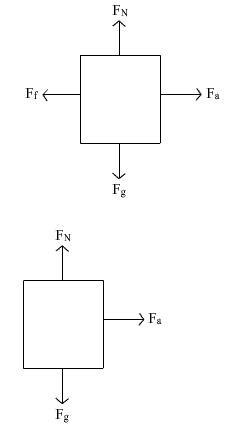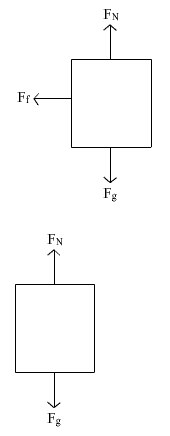Detailed Solution for Test: Free Body - 2 - Question 4

For a body to move with constant velocity the net force on body should be zero.Only option A can have a net force zero rather b,c net horizontal force is non-zero and in option d there is no horizontal force so no motion in horizontal direction So option a is most appropriate

Test: Free Body - 2 - Question 5

A lift of mass 1000kg , which is moving with an acceleration of 1 m/s2 in upward direction has tension developed in its string equal to:

Detailed Solution for Test: Free Body - 2 - Question 5

The mass of the lift is 1000kg, the acceleration is 1m/s2 and the acceleration due to gravity is 9.8m/s2.

The tension developed in string is given as,

T = mg+ma

= 1000×9.8+1000×1

= 10800N

Thus, the tension developed in the string is 10800N.

Test: Free Body - 2 - Question 6

25 N force is required to raise 75 kg mass from a pulley. If rope is pulled 12 m , then the load is lifted to 3 m , the efficiency of pulley system will be:

Detailed Solution for Test: Free Body - 2 - Question 6

Efficiency = output/input = (75×3) / (25×12)

=75/100 =75%

Test: Free Body - 2 - Question 7

An ice cart of mass 60 kg rests on a horizontal snow patch with coefficient of static friction 1/3 . Assuming that there is no vertical acceleration , find the magnitude of maximum horizontal force required to move the ice cart.

Detailed Solution for Test: Free Body - 2 - Question 7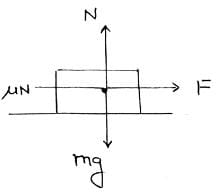Let F be the min. force required to move the cart
then F = μN (along x axis )
Δ N = mg (along y x axis )
⇒ F = μmg = 1/3 x 60 x 9.8
⇒ F = 196 N.

Test: Free Body - 2 - Question 8

A mass of 100 kg is resting on a rough inclined plane of 60o. If the coefficient of friction is 0.5, then the least force acting parallel to the plane to keep the mass in equilibrium is

Detailed Solution for Test: Free Body - 2 - Question 8

The force parallel to the plane but downwards, W = mg.sin 60 = 980 x √3/2 = 848.7N
Maximum friction force acting, f = mg.cos 60 x 0.5 = 980 x ½ x 0.5 = 245N
Thus the minimum extra force required, let say F = 848.7 - 245 = 603.3 N

Test: Free Body - 2 - Question 9

Three blocks connected together lie on a horizontal frictionless table and pulled to the right with a force of F = 100 N. If m1 = 10 kg, m2 = 20 kg, m3 = 30kg. Then the tension T1 and T2 will be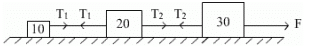Detailed Solution for Test: Free Body - 2 - Question 9

Considering the three block system, we have
100 = 60.a
where a is the common acceleration of all the blocks
a = 5/3 m/s2
Considering mass 1 we get,
T1 = 10 a
T1 = 50/3 N
Considering mass 1 and 2 we get,
T2  = 30 a
T2 = 50N

Test: Free Body - 2 - Question 10

Block A has mass 10 kg and block B has mass 5 kg. They are connected by an inextensible rope which passes over the mass less and frictionless pulley. Select the correct free body diagrams for two blocks from the given options.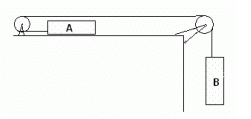Detailed Solution for Test: Free Body - 2 - Question 10

On the block A, the tension acts leftwards while friction acts rightwards due to normal upwards which balances weight downwards. Similarly the tension acts upwards on block B which balances its weight.

## Physics Class 11

126 videos|449 docs|210 tests
 Use Code STAYHOME200 and get INR 200 additional OFF Use Coupon Code
Information about Test: Free Body - 2 Page
In this test you can find the Exam questions for Test: Free Body - 2 solved & explained in the simplest way possible. Besides giving Questions and answers for Test: Free Body - 2, EduRev gives you an ample number of Online tests for practice

## Physics Class 11

126 videos|449 docs|210 tests

### How to Prepare for NEET

Read our guide to prepare for NEET which is created by Toppers & the best Teachers